# Schwarz integral

A parameter-dependent integral that gives a solution to the Schwarz problem on expressing an analytic function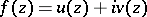in the unit discby the boundary values of its real (or imaginary) parton the boundary circle(see ).

Let on the unit circle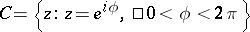a continuous real-valued function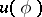be given. Then the Schwarz integral formulas defining an analytic function, the boundary values of whose real part coincide with(or the boundary values of whose imaginary part coincide with), have the form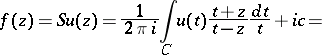(*)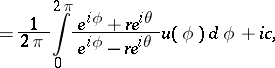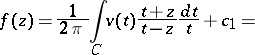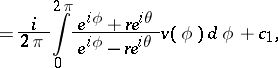where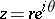,, andand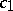are arbitrary real constants. The Schwarz integral (*) is closely connected with the Poisson integral. The expression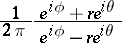is often called the Schwarz kernel, and the integral operatorin the first formula of (*) is called the Schwarz operator. These notions can be generalized to the case of arbitrary domains in the complex plane (see ). The Schwarz integral and its generalizations are very important when solving boundary value problems of analytic function theory (see also ) and when studying boundary properties of analytic functions (see also ).

When applying the integral formulas (*), a very important and more difficult problem arises concerning the existence and the expression of the boundary values of the imaginary part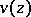and of the complete functionby the given boundary values of the real part(or of expressing the boundary values of the real partand those of the complete functionby the given boundary values of the imaginary part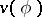). If the given functionsorsatisfy a Hölder condition on, then the corresponding boundary values oforare expressed by the Hilbert formulas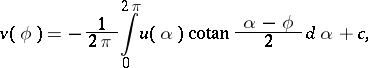here the integrals in these formulas are singular integrals and exist in the Cauchy principal-value sense (see , and also Hilbert singular integral).